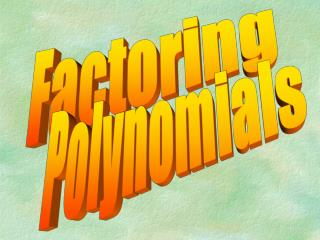DownloadDownload PresentationFactoring

# Factoring

Download Presentation## Factoring

- - - - - - - - - - - - - - - - - - - - - - - - - - - E N D - - - - - - - - - - - - - - - - - - - - - - - - - - -
##### Presentation Transcript

1. Factoring Polynomials

2. Factoring Method #1 Factoring polynomials with a common monomial factor (using GCF). **Always look for a GCF before using any other factoring method.

3. Steps: 1. Find the greatest common factor (GCF). 2. Divide the polynomial by the GCF. The quotient is the other factor. 3. Express the polynomial as the product of the quotient and the GCF.

4. Factor Each Polynomialwe will use the opposite of the “box method” 1. 24x + 48y 24x 48y

5. 2. 9x2 – 3x 9x2 -3x

6. 3. 64 – 40ab 64 -40ab

7. 5. 30mn2 + m2n – 6n

8. 6. x4 – 18x3 + 22x

9. 7. 14y3 – 28y2 + y

10. Solve Each Equation • Set each piece equal to zero • Solve for all pieces

11. 1. x(x – 32)

12. 2. 4b(b + 4)

13. 3. (y – 3)(y + 2)

14. 4. 8p2 – 4p = 0

15. 5. 2m2= -20m